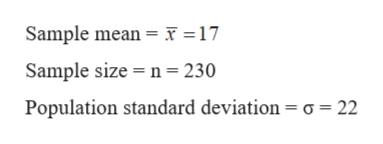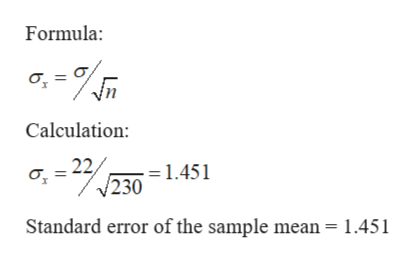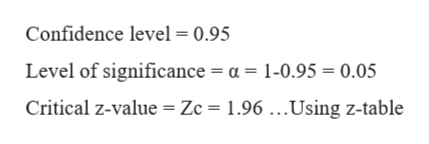# A sample of 230 observations is selected from a normal population with a population standard deviation of 22. The sample mean is 17.a) Determine the standard error of the mean. (Round your answer to 3 decimal places.)  b) Determine the 95% confidence interval for the population mean. (Use z Distribution Table.) (Round your answers to 3 decimal places.)confidence interval _____________ and______________

Question
33 views

A sample of 230 observations is selected from a normal population with a population standard deviation of 22. The sample mean is 17.

a) Determine the standard error of the mean. (Round your answer to 3 decimal places.)

b) Determine the 95% confidence interval for the population mean. (Use z Distribution Table.) (Round your answers to 3 decimal places.)

confidence interval _____________ and______________

check_circle

Step 1

Given:help_outlineImage TranscriptioncloseSample mean F = 17 Sample size n= 230 Population standard deviation = o 22 fullscreen
Step 2

a)

Standard error of the sample mean:help_outlineImage TranscriptioncloseFormula Calculation: 22 = 1.451 230 Standard error of the sample mean = 1.451 fullscreen
Step 3

b) To find 95% confidence interval for the p...help_outlineImage TranscriptioncloseConfidence level = 0.95 Level of significance = a = 1-0.95 = 0.05 Critical z-value = Zc = 1.96 ...Using z-table fullscreen

### Want to see the full answer?

See Solution

#### Want to see this answer and more?

Solutions are written by subject experts who are available 24/7. Questions are typically answered within 1 hour.*

See Solution
*Response times may vary by subject and question.
Tagged in

### Hypothesis Testing